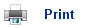# Born-Haber Cycles

• The lattice enthalpy for a compound is defined as the enthalpy change when 1 mole of a solid in its standard state is formed from its ions in the gaseous state. For example,

Na+(g) + Cl-(g)NaCl(s)

• Lattice enthalpies for ionic compounds give a good indication as to the strength of the ionic bonding in the lattice.
• Lattice enthalpies cannot be measured directly; they are calculated indirectly using a Born-Haber cycle.
• This splits up the enthalpy of formation into individual enthalpy changes; one of which is the lattice enthalpy. For example, the above reaction can be split up into several steps:
• The elements in their standard states are turned into gaseous atoms.
• The gaseous atoms become gaseous ions.
• The gaseous ions come together to form solid sodium chloride.
• By applying Hess’s Law, the lattice enthalpy can then be calculated.
• The following enthalpy changes are often used in Born-Haber cycles: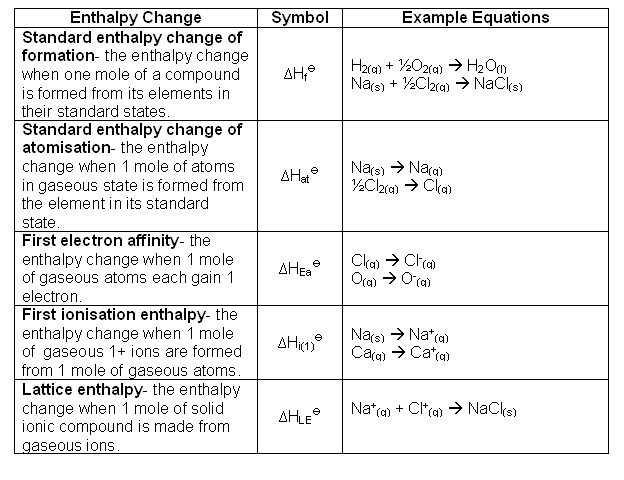• At each stage of the Born-Haber scale, one change occurs.
• The following shows an example Born-Haber cycle: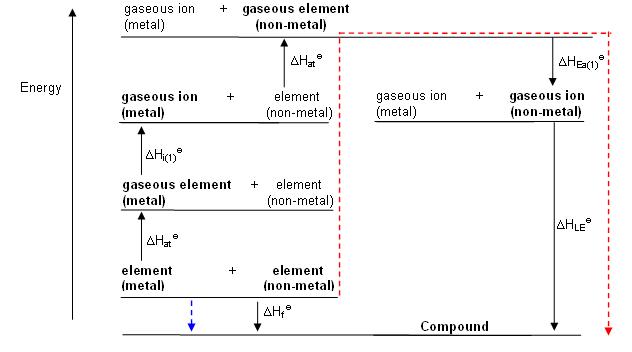• Applying Hess’s law to this cycle, which states that the enthalpy change for a reaction is the same regardless of how many steps occur in that reaction, it is possible to calculate the lattice enthalpy.
• The arrows pointing upwards represent endothermic changes, those pointing downwards show exothermic changes.
• From the Born-Haber Cycle, the enthalpy change associated with the route depicted by the blue arrow is equal to the enthalpy change of the route shown by the red arrow.
• The Born-Haber cycle for sodium chloride is shown below: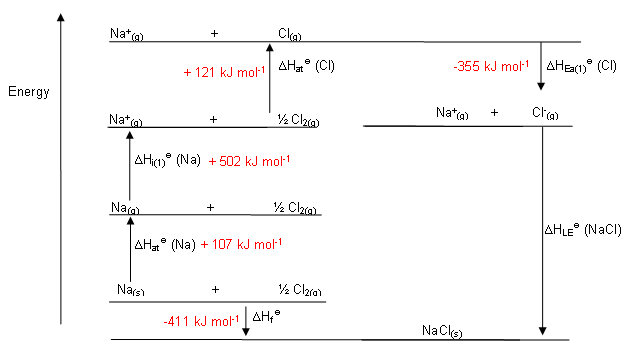• So, from the cycle: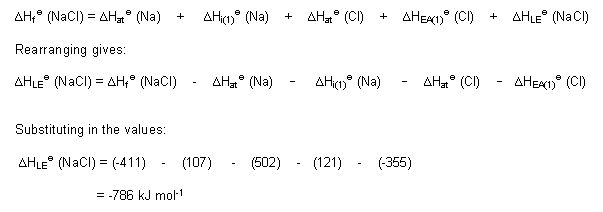## Useful books for revision

Revise A2 Chemistry for Salters (OCR A Level Chemistry B)Salters (OCR) Revise A2 ChemistryHome